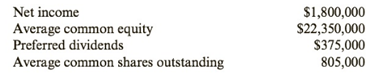Chapter 10, Problem 37CE### Cornerstones of Financial Accounti...

4th Edition
Jay Rich + 1 other
ISBN: 9781337690881

#### Solutions### Cornerstones of Financial Accounti...

4th Edition
Jay Rich + 1 other
ISBN: 9781337690881
Textbook Problem
3 views

# Stockholder Profitability RatiosThe following information pertains to Montague CorporationRequired:Calculate the return on common equity and the earnings per share. (Note: Round answers to two decimal places.)

To determine

Concept introduction:

Return on Equity:

Return on Equity is the rate of return earned by the Stockholders on their investment in the company. It is calculated with the help of following formula:

Return on Equity = Net IncomeAverage Stockholders Equity

The Average stock holder’s equity calculated with the help of following formula:

Average stock holders equity=( Beginning stock holders equity + Ending stock holders equity)2

Basic Earnings per share:

The Basic Earnings per share is the amount of net income earned by each common share outstanding. The Earnings per share calculated by with help of following formula:

Basic Earnings per share=Net Income - Preferred DividendWeighted Average Common Shares Outstanding

Net Income available to common stockholder = Net income  Preferred Dividend

To calculate:

The Return on Equity and Earnings per share.

Explanation

The Return on Equity and Earnings per share are calculated as follows:

 Net Income (A) $1,800,000 Average Common Equity (B)$ 22,350,000 Preferred Dividends (C) \$3...

### Still sussing out bartleby?

Check out a sample textbook solution.

See a sample solution

#### The Solution to Your Study Problems

Bartleby provides explanations to thousands of textbook problems written by our experts, many with advanced degrees!

Get Started

#### Find more solutions based on key concepts